##### MRU-120HD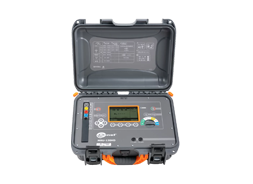• Measurement of resistance of auxiliary electrodes RS and RH
• Measurement of interference voltage
• Measurement in the presence of interference voltage generated by power networks with frequency of 50 Hz or 60 Hz
##### MRU-11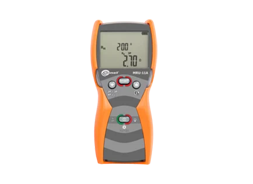• Measurement of resistance of auxiliary electrodes RS and RH
• Measurement of interference voltage to 100 V
• Selection of maximum measuring voltage (25 V and 50 V)

##### MRU-120 XL3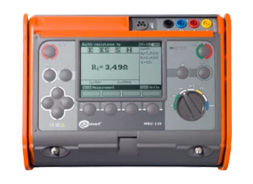• Measurement current of 200 mA facilitates earth resistance measurements in difficult areas
• Measurement of resistance of auxiliary electrodes RS and RH
• Measurement of interference voltage to 100 V
##### MRU-120 XL3N1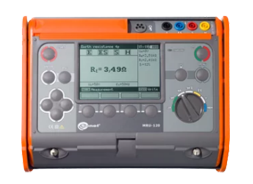• Measurement current of 200 mA – facilitates earth resistance
• Measurement of resistance of auxiliary electrodes RS and RH
• Measurement of interference voltage
##### MRU-200 XL3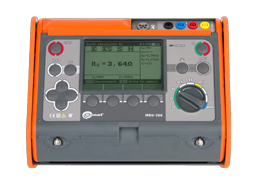• Measurement of resistance of auxiliary electrodes RS and RH
• Measurement of interference frequency
• Measurement of interference voltage
##### MRU-200 XL3C3N1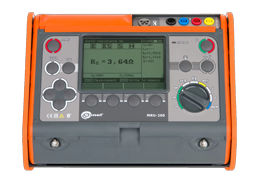• Measurement of resistance of auxiliary electrodes RS and RH
• Measurement of interference frequency
• Measurement of interference voltage
##### MRU-200-GPS XL3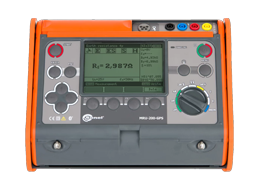• Measurement of resistance of auxiliary electrodes RS and RH
• Measurement of interference frequency
• Measurement of interference voltage
##### MRU-200-GPS XL3C3N1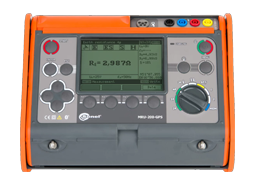• Measurement of resistance of auxiliary electrodes RS and RH
• Measurement of interference frequency
• Measurement of interference voltage
##### MRU-200-GPS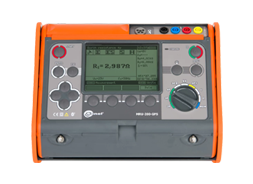• Measurement of resistance of auxiliary electrodes RS and RH
• Measurement of interference frequency
• Measurement of interference voltage
##### MRU-200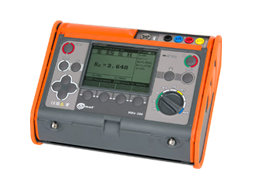• Measurement of resistance of auxiliary electrodes RS and RH
• Measurement of interference voltage
• Measurement in the presence of interference voltage generated by power networks with frequency of 16 2/3 Hz, 50 Hz, 60 Hz, 400 Hz
##### MRU-120• Measurement of resistance of auxiliary electrodes RS and RH
• Measurement of interference voltage
• Measurement current of 200 mA – facilitates earth resistance measurements in difficult areas (sand, stony soil)
##### MRU-30• Measurement of resistance of auxiliary electrodes RS and RH
• Measurement of interference voltage
• Measurement in the presence of interference voltages in networks with frequencies of 50 Hz and
60 Hz
##### MRU-21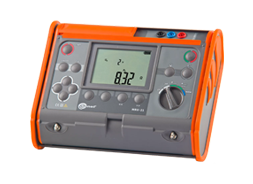• Measurement of resistance of auxiliary electrodes RS and RH
• Measurement of interference voltage
• Selection of maximum measuring voltage (25 V and 50 V)
##### MRU-10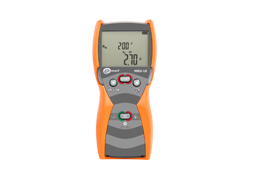• Measurement of resistance of auxiliary electrodes RS and RH
• Measurement of interference voltage
• Selection of maximum measuring voltage (25 V and 50 V)